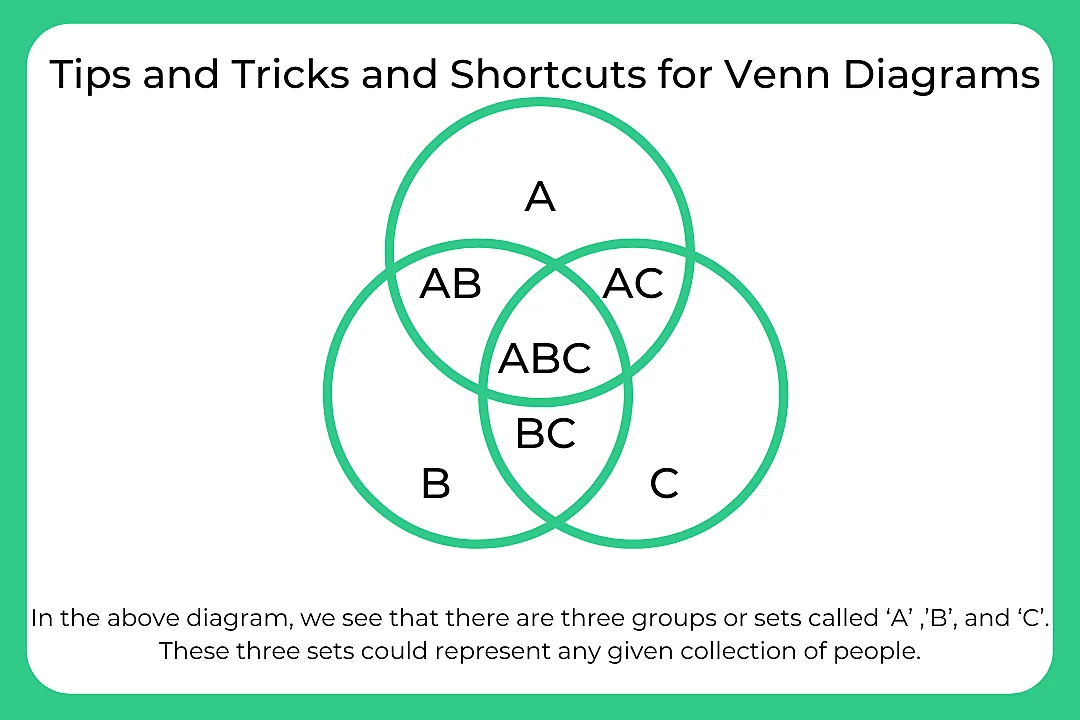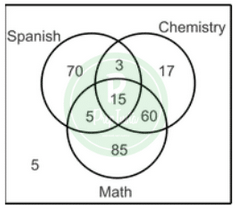# Tips And Tricks And Shortcut For Venn Diagrams

## Tips and Tricks for Venn Diagrams

Venn Diagrams are a schematic and identical way of representing the elements of a set or a group. In mathematical language, it represents the intersection of the two groups. So accordingly, Venn Diagrams are quite important in both quantitative and logical reasoning. Therefore, it is important to practice Venn Diagrams Questions along with the Tips and Tricks for Venn Diagrams.For example –  A contains all the people that like cake. Set B represents all the people who like ice cake and set C represents all the people who like chips. Then the region marked as AB represents all the people who like both cake and ice cake. The region marked BC represents all the people who like both ice cake and chips. Similarly, the region AC represents all the people who like cake and ice cake.### Related Banners

Get PrepInsta Prime & get Access to all 200+ courses offered by PrepInsta in One Subscription

If there are only two elements

n ( A ∪ B) = n(A ) + n ( B ) – n ( A∩ B)

If there are three elements

n (A ∪ B ∪ C) = n(A ) + n ( B ) + n (C) – n ( A ∩ B) – n ( B ∩ C) – n ( C ∩ A) + n (A ∩ B ∩ C)

### Question 1

In a class of 260 seniors, 93 study Spanish, 95 study Chemistry, 165 study Mathematics, 18 study Spanish and Chemistry, 75 study Chemistry and Math, 20 study Math and Spanish and 15 study all three subjects. Make a Venn diagram to illustrate the data and then find the probability that a student selected at random studies Spanish but not Maths.Options

(a) 0.121
(b) 0.0192
(c) 0.269
(d)0.387

Correct Option (A)

Explanations

•  Spanish  but not Maths and Chemistry

P(S∩M’∩C’) = $\frac{70}{260}$ = $\frac{7}{26}$ = 0.269

• Maths and Chemistry but not Spanish

P(MCS)= $\frac{60}{230}$ = $\frac{3}{13}$ = 0.231

• None of these subject

P(MCS) = $\frac{5}{260}$ = $\frac{1}{52}$

• Spanish, given that he/she studies Math

P(S, M)$\frac{P(S\cap M')}{P(M)} = \frac{\frac{20}{260}}{\frac{165}{260}} = 0.121$

### Question 2

Given P(A∩B) = 0.4, P(A∩B’) = 0.2 and P(A’∩B’) = 0.3, find P(B) and P(A|B).Options

(a) 0.8
(b) 0.6
(c) 0.4
(d) 0.2

Correct Options (A)

Explanations

The missing value, P(B∩A’), must be 0.1 in the order for the total of the probabilities in the sample space equal to 1. Thus , P(B) = 0.5
P $\frac{A}{B} = \frac{P(A∩B)}{P(B)} \$ = $\frac{P(0.4)}{P(0.5)} \$ = 0.8

## Get over 200+ course One Subscription

Courses like AI/ML, Cloud Computing, Ethical Hacking, C, C++, Java, Python, DSA (All Languages), Competitive Coding (All Languages), TCS, Infosys, Wipro, Amazon, DBMS, SQL and others

## Get over 200+ course One Subscription

Courses like AI/ML, Cloud Computing, Ethical Hacking, C, C++, Java, Python, DSA (All Languages), Competitive Coding (All Languages), TCS, Infosys, Wipro, Amazon, DBMS, SQL and others